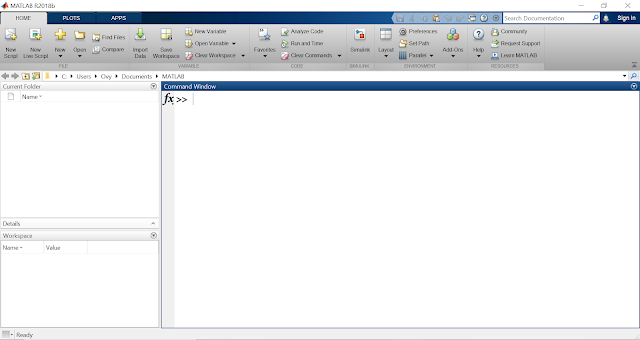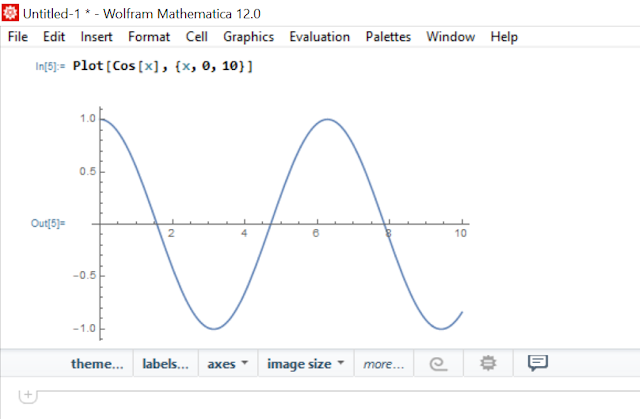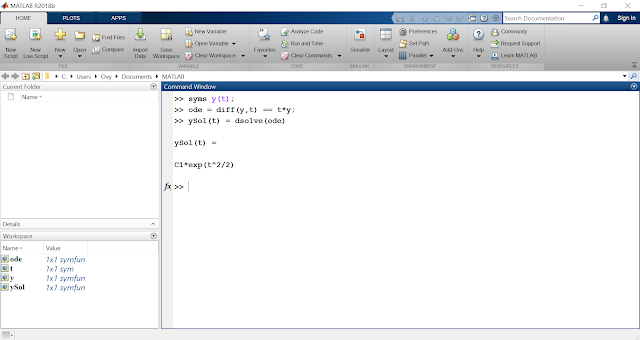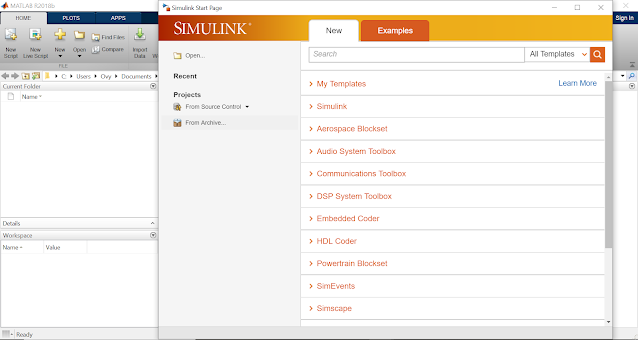## Welcome to the World of Modelling and Simulation

### What is Modelling?

This blog is all about system dynamics modelling and simulation applied in the engineering field, especially mechanical, electrical, and ...### MATLAB vs Mathematica: Which one is Better?

There is a long debate between MATLAB and Mathematica fans. Someone prefers one over another and thinks that it is better than the other and starts the battle. Both MATLAB and Mathematica are used for mathematical computations, and they are written in C, C++, Java, and their own proprietary languages. I am not going to say, which one is better. Rather, I am going to highlight some facts as an average user of both these tools.

At first, look at their opening windows below. MATLAB has more menus/options in their welcoming interface, whereas Mathematica has a very plain and simple background.MATLAB WindowMathematica Window

Now, let's look at how difficult or easy it is to plot a very simple function in both Mathematica and MATLAB. Below, we see that a Cosine plot is created by these tools. We are plotting a function where the range is defined from 0 to 10, and everything remains exactly same in both tools for comparison. We see that Mathematica needs a single line to generate the plot, whereas MATLAB takes 3 lines to plot. Moreover, the plot in Mathematica looks smoother and sharper than the MATLAB. The reason is, for MATLAB to generate such level of smoothness, we need to divide the range (0 - 10) into more points, which needs to be done manually. It is also noticeable that Mathematica displays the plot right underneath the command line, but MATLAB does that by opening another figure window.Plot in MathematicaPlot in MATLAB

Let's solve an ordinary differential equation (ODE) symbolically using both software. Assume a very simple first order ODE, dy/dt = ty. Since no initial condition is specified, the general solution of this ODE will have a constant term. To solve it symbolically, from the images below, we see that MATLAB takes 3 lines at the command prompt to produce the solution. On the other hand, Mathematica again takes a single line to solve the problem.Differential Equation Solution in MATLAB SymbolicallyDifferential Equation Solution in Mathematica Symbolically

So far, we have seen that it takes less effort (considering the number of command lines) in Mathematica to do the same job than MATLAB. But, those who are proficient in MATLAB, they can write these commands at greater speed and efficiency; therefore, it actually does not matter that much. Also, it is visible that both software have similar command styles with little syntax changes, for example, in MATLAB, the command to generate a graph is 'plot' with a small 'p', whereas in Mathematica, it is 'Plot' with a capital 'P'. Similarly, 'dsolve' in MATLAB is written 'Dsolve' with a capital 'D' in Mathematica. This implies that if anybody knows any of these tools, then it is easier for them to learn the other.

In the end, I would like to summarize the facts that both MATLAB and Mathematica have similar features and usabilities in mathematical computations. But, the style of coding is different although there are minute disparities in basic commands. Nevertheless, let's not forget the fact that MATLAB is a huge platform not only for the basic mathematical computations, but also it has vast implementations in numerous fields, such as, control engineering, signal processing, telecommunication, vibration and dynamics analyses, and so on. MATLAB has an integrated tool called SIMULINK, which has many libraries to handle different varieties of tasks. Also, they are done graphically in SIMULINK. Below, is a screenshot of SIMULINK in MATLAB.Showing SIMULINK in MATLAB environment with different libraries